# Lewis structures

### Lewis structures

#### Lessons

In this lesson, we will learn:
• To construct Lewis (dot and cross) diagrams by applying our knowledge of electrons and bonding.
• To explain the difference between ionic and covalent compounds using Lewis diagrams.

Notes:
• Molecules and compounds alike are held together by chemical bonds between their constituent atoms - the atoms they're made from. These are the intramolecular (ionic and covalent) bonds we saw earlier.

• Chemical bonds can be represented using Lewis structures, also known as dot-and-cross diagrams, which show the outer shell electrons of all the atoms in a molecule forming the bonds holding it together.

• In Lewis structures, dots and crosses are used to show electrons in the outer shells, where one of the two are used for the central atom and the other used for the non-central atom(s) it is bonding to.

• REMEMBER: You don't need to draw inner electron shells in Lewis structures!

• They can be used to show covalent and ionic bonding between atoms in molecules and compounds.

• When drawing these diagrams, recall the lesson on Electronic structure: 288 rule for help when finding the number of outer shell electrons.

• Lewis structures for a covalent compounds can be drawn like this (see the diagrams for examples of CO$_2$ and H$_2$O):
• Step 1: Write the chemical symbol for the central atom in the molecule and draw a ring around it. If the molecule is diatomic, draw either one atom.
• Example 1:Example 2:• Step 2: Write the symbols of the other atoms, equally spaced around it. Draw a ring around each of these so that their rings each have an overlap into the central ring. These rings are the outer electron shells.
• Example 1:Example 2:• Step 3: For the non-central atoms, fill in their outer shells of electrons using the 288 rule learned in Lesson 2.5 (Electronic structure in atoms). Remember to fill up to four electrons individually then start pairing them up. Mark all of these electrons using either a dot or a cross. Be consistent with the dots and crosses – e.g. if you use crosses for one H atom, use it for all H atoms.

• Example 1: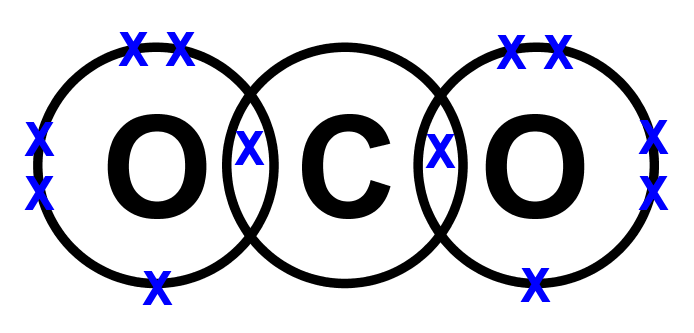Example 2: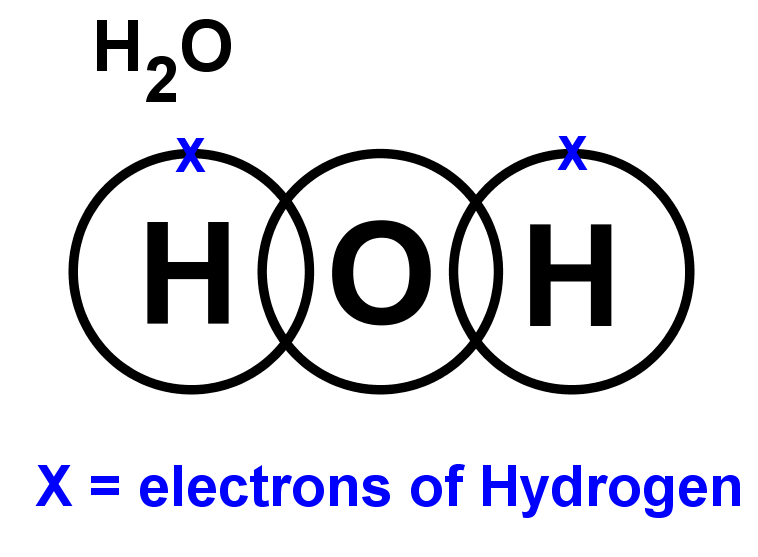• Step 4: LOOK CAREFULLY AT YOUR DIAGRAM. Take a moment and make sure any unpaired electrons are placed in the overlap with the central atom.

• Example 1: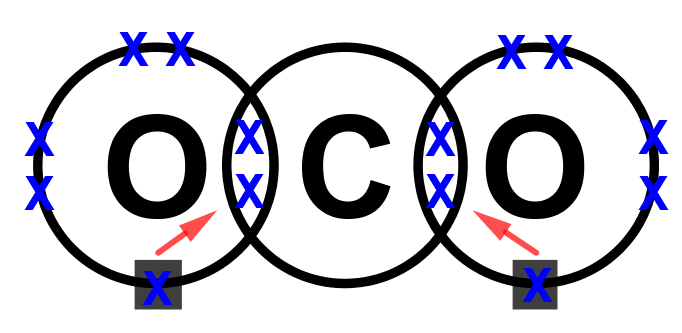Example 2: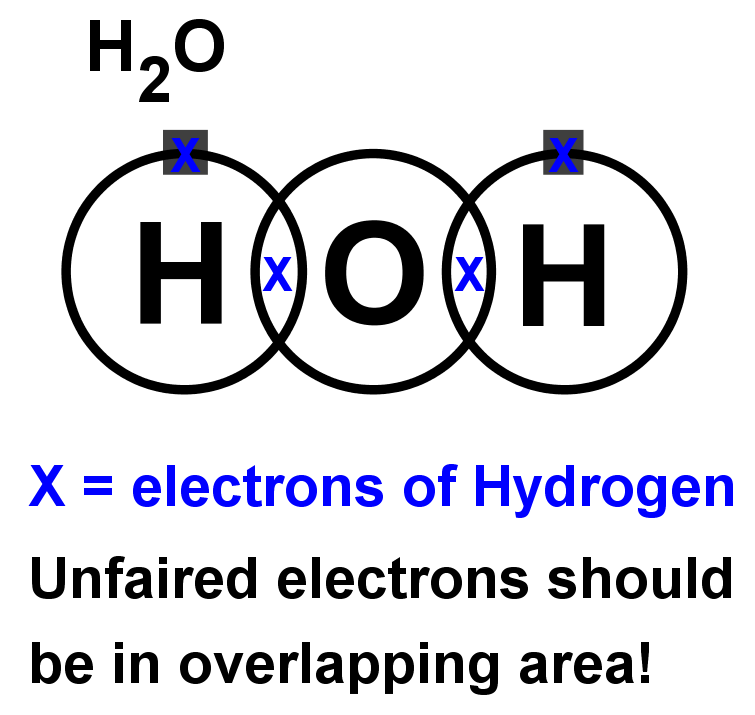• Step 5: Now fill the outer shell of electrons in the central atom. Use the other sign to the one you used with the non-central atom. Start by making pairs of dots and crosses with the electrons of the non-central atoms in the overlapping area. You can only pair dots and crosses in a 1:1 ratio. Now, any extra electrons can be placed outside the overlaps on the outer shell. Remember that if there are more than four electrons in the shell they must now be paired. Electrons in the overlapping area count for both overlapping atoms!

• Step 6: Look carefully at your diagram,. If you have followed these steps, all of your atoms should have exactly 8 electrons (2 if it is hydrogen) in the outer shell. Remember that electrons in the overlaps count for both atoms.

• Example 1: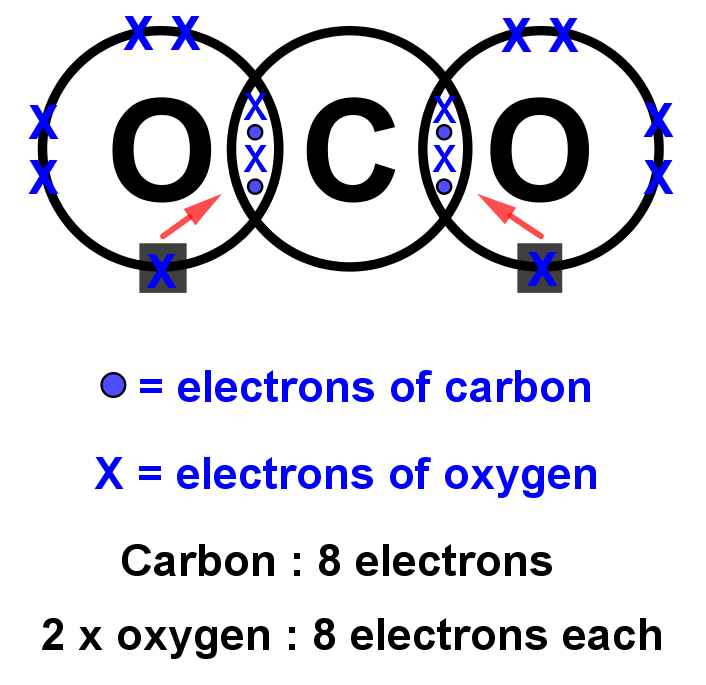Example 2: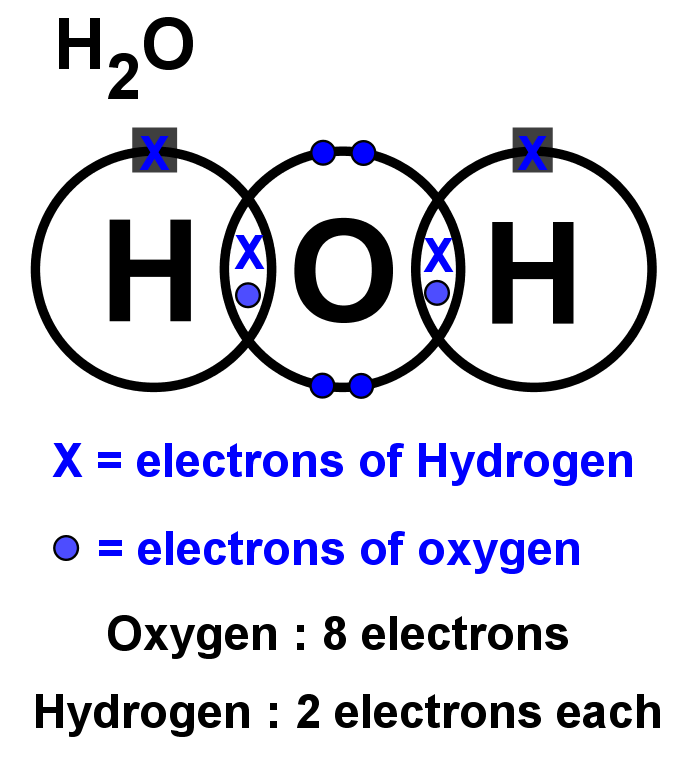• Dot and cross diagrams for ionic compounds can be drawn like this:
• Step 1: Write the chemical symbols of the atoms side by side and draw a ring around them but keep them separate. They must not overlap like in covalent compounds.

• Step 2: Fill the outer shell with the correct number of electrons for each atom.

• Example: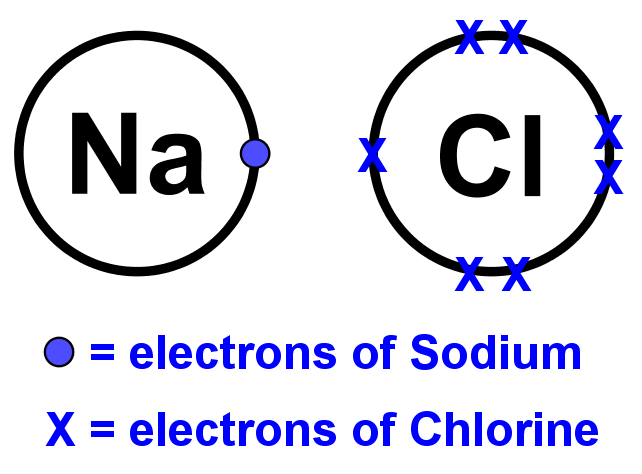• Step 3: Move the electrons from the metal atom(s) to the non-metal atom(s). This should complete the outer shell of the non-metal atom(s) while leaving empty the outer shell of the metal atom(s).

• Example: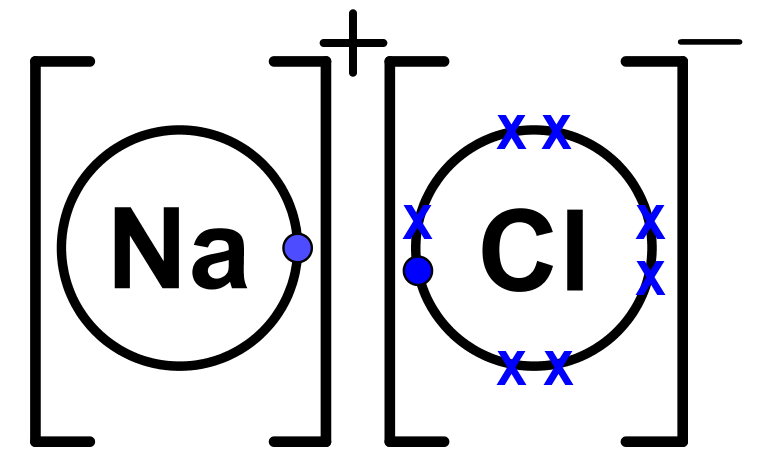• These diagrams should help to clarify the difference between bonding in covalent and ionic compounds:
• Covalent bonding involves the sharing of electrons to complete outer shells in both atoms.
• Ionic bonding involves a complete transfer of electrons usually from a metal atom to a non-metal atom, which also leads to complete outer shells.
• Introduction
Introduction to dot and cross diagrams
a)
How do we show atoms bonding?

b)
Lewis diagram: a walkthrough.

c)
Different ways of bonding.

d)
Exceptions to the octet rule.

• 1.
Draw Lewis structures for simple covalent compounds.
Draw dot and cross structures for the following molecules:
a)
HCl

b)
N$_2$

c)
NH$_3$

d)
AlCl$_3$

e)
NH$_{4}$+

• 2.
Draw Lewis structures for simple ionic compounds.
Draw dot and cross structures for the following molecules:
a)
KCl

b)
Na$_2$O

c)
CaCl$_2$